Blogging Fusion Blog Directory the #1 blog directory and oldest directory online.

## Unifying Quantum and Relativistic Theories

Home Unifying Quantum and Relativistic Theories

## Unifying Quantum and Relativistic Theories

Rated: 4.22 / 5 | 975 listing views• Jeffrey O'Callaghan
• January 15, 2020 05:43:57 PM

The purpose of this blog is to show how changing ones perspective on the physical structure of the universe will allow one to unite the abstract concepts of Quantum Mechanics with the observable realities of both Einstein's Special and General Theories of Relativity.

## Listing Details

• Website Tags:
• Annual Regular Membership: 1 Year Term 2021-01-15 17:43:57 (43 days left)
• ## Listing Statistics## Alexa Web Ranking: 4,379,434## Example Ad for Unifying Quantum and Relativistic Theories

This what your Unifying Quantum and Relativistic Theories Blog Ad will look like to visitors! Of course you will want to use keywords and ad targeting to get the most out of your ad campaign! So purchase an ad space today before there all gone!

https://www.bloggingfusion.com

.

• Place a detailed description
• It appears here within the content
• Approved within 24 hours!
• 100% Satisfaction
• Or 3 months absolutely free;

## Subscribe to Unifying Quantum and Relativistic Theories

The errors in the Big Bang Theory

Please follow and like us:0.9k1.1k7884041kThe Big Bang Theory is the leading explanation about how the universe began. At its simplest, it says the universe as we know it started with a small singularity, then inflated over the next 13.8 billion years to the cosmos that we know today. Because current instruments don’t allow astronomers to […] The post The errors in the Big Bang Theory appeared first on Unifying Quantum and Relativistic...

The Big Bang Theory is the leading explanation about how the universe began. At its simplest, it says the universe as we know it started with a small singularity, then inflated over the next 13.8 billion years to the cosmos that we know today.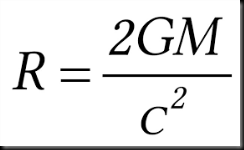Because current instruments don’t allow astronomers to peer back at the universe’s birth, much of what we understand about the Big Bang Theory comes from mathematical formulas and models. Astronomers can, however, see the "echo" of the expansion through a phenomenon known as the cosmic microwave background.

The idea the universe was smaller in the beginning was supported by Edwin Hubble in 1929 it expanding.

Later, a few physicists led by George Gamow a proponent of the big bang model showed an expanding universe meant that it might have had its beginning in a very hot infinitely dense environment, which then expanded to generate the one we live in today.

They were able to show only radiation emitted approximately 300,000 years after the beginnings of the expansion should be visible today because before that time the universe was so hot that protons and electrons existed only as free ions making the universe opaque to radiation. This period is referred as the age of "recombination".

Additionally, they predicted this Cosmic Background Radiation or what was left over from the age of recombination would have cooled form several thousand degrees Kelvin back when it was generated to 2.7 today due to the expansion of the universe.  Many thought its discovery 1965 by Penzias and Wilson provided its verification

However, there was a problem with assuming the universe begin as an expansion of in an infinitely dense hot environment because one would expect it and the Cosmic Background Radiation to be homogeneous because an infinitely dense environment must have been, by definition homogeneous. Therefore, if the universe was homogeneous when it began it should still be.

But the existence of galactic clusters and the variations in the intensity of the cosmic background radiation discovered by NASA’s WMAP satellite showed the universe is not and therefore, was not homogeneous either now or at the time when the Cosmic Background Radiation was emitted.

Many proponents of the big bang model assume that these "anisotropy" in the universe are caused by quantum fluctuations in the energy density of space. They define quantum fluctuations as a temporary change in the energy of space caused by the uncertainty principle.

However, there is an error in the math used to predict both effects the expansion of singularity at its origin and quantum fluctuations in the energy density of space would have on the evolution of the universe.

Einstein mathematics tell us time slows as the gravitational or energy density increases and will eventually stop if it becomes great enough.  While observation of black holes provides verification of his math because it is observed that time does slow to a stop when it reaches a critical energy density at its event horizon.  Additionally, Schwarzschild was able to use Einstein’s math to calculate the radius of a black hole were the energy density would be great enough to stop time.

This means the math used by the proponents of the big bang is INCORRECT if they did not include the effect the energy density around a singularity or quantum fluctuation would have on its evolution.

This is because observationally verified math of Schwarzschild tells us there is a minimum radius the total energy content of the universe can occupy for time to move forward. Since evolution cannot occur in an environment where time has stopped that is also MINIMUM RADIUS of the universe which could expand form which IS larger than a singularity.

In other words, if they had included the effect energy density has on time, they would have realized that the universe could not have originated from a singularity.

Some may say that the energy density of expanding universe would not effect the rate at which time passes but they would be wrong because Einstein’s tells us it is only related to differential energy density. In other words, he tells us the rate at which time slows and where it would stop and prevent further expansion would be determined by the differential energy density between the center of its expansion and its outer edge.  This point would define the minimum volume it would have to have before its expansion could take place.

However, there is a similar error in the math behind the assumption that quantum fluctuations are responsible for "anisotropy" in Cosmic Background Radiation because energy could not expand from one because the energy density surrounding it would cause time to stop.  Therefore, quantum fluctuation could not affect the evolution of the universe or be responsible for "anisotropy" in Cosmic Background Radiation because as was just mentioned evolution cannot occur in an environment where time has stopped.

Some might disagree because they say the energy in a singularity and that contained in a quantum fluctuation would be powerful enough to overcome the stopping of time predicted by Einstein mathematics.  However, they would be wrong because the mathematics of Einstein tells that when the energy density reaches a certain level time will stop.  It does not say that an increase beyond that point will allow time to move again.

As was mentioned earlier, current instruments don’t allow astronomers to peer back at the universe’s birth, much of what we understand about the Big Bang Theory comes from mathematical formulas and model

However, we may be able to define the origin of the present universe in terms of its observable properties.

We still have not been able to determine if the universe will continue to expand indefinitely or if it will eventually collapse in on itself. However, if one assumes it does, we could develop a mathematically model which would tell us when the heat generated by its collapse would be enough to cause it to re-expand.  Additionally, one could determine if that heat occurred AFTER that required to free protons and electrons from each other thereby allowing another age of "recombination" when it started to re-expand.

This would also give mathematicians the ability to more precisely determine the age of universe because we can observe when age of "recombination" occurred and project back from that point in time to when the additional heat generated by its continued collapse was great enough to cause it to re-expand.

In others words we have the ability to define the origin of the present universe and anisotropy" in Cosmic Background Radiation in terms of a mathematical model based on real time observations of the present universe.

The science of Astrophysics is base almost exclusively on observations.  Therefore, the question they must ask themselves is "If we have two models for the origin of the universe that predict the same outcome which one should we assume is correct?"  The one that make is predictions based on the observable properties of our present universe or one that defines it origins in terms of the unobservable properties of a singularity.

<font face="Arial">New Page 1</font><font face="Arial">New Page 1</font>

<font face="Arial">New Page 1</font>Please visit our  Facebook group The Road to unification of Quantum and Relativistic theories if you would like to comment or contribute to our project e Road to unification part  2007 thru 2010  Ebook

 The Road to Unifying QM with Relativity part 1 2007 thru 2010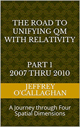Ebook \$8.00 Paper Back \$15.00 The Road to Unifying QM with Relativity part 2 2011 thru 2014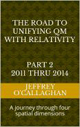Ebook \$8.00 Paper Back \$16.00 The Road to Unifying QM with Relativity part 3 2015 thru 2020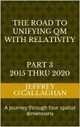Ebook \$8.00 Paper Back \$18.00

The post The errors in the Big Bang Theory appeared first on Unifying Quantum and Relativistic Theories.

Incorporating gravity into Quantum electrodynamics

Quantum electrodynamics (QED) is the relativistic quantum field theory of electrodynamics.  In essence, it describes how light and matter interact and is the first theory where full agreement between quantum mechanics and special relativity is achieved. QED mathematically describes all phenomena involving electrically charged particles interacting by means of exchange of photons and represents the […] The post Incorporating gravity into Quantum electrodynamics appeared first on...

Quantum electrodynamics (QED) is the relativistic quantum field theory of electrodynamics.  In essence, it describes how light and matter interact and is the first theory where full agreement between quantum mechanics and special relativity is achieved. QED mathematically describes all phenomena involving electrically charged particles interacting by means of exchange of photons and represents the quantum counterpart of classical electromagnetism giving a complete account of matter and light interaction.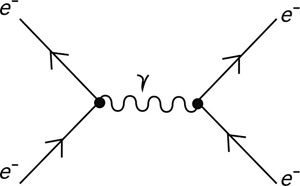However, as of yet no one has been able to integrate gravity into its theoretical structure.

Yet one can use the wave properties of a quantum field to explain how Einstein’s definition of gravity in his General Theory of Relativity can be used to accomplish that.

Einstein in his General Theory of Relativity explains gravity as a distortion of space-time caused by the presence of matter or energy while defining its magnitude in terms of the concentration of matter or energy in a given volume of space-time

Therefore, to incorporate Quantum field into Einstein General Theory of Relativity one must explain how the interaction of a photon with electrically charge particles causes a distortion in space-time associates with gravity.

As was mentioned earlier QED defines the interaction of charged particles in terms of the exchange of photons.  However, it defines the exchange of photons in terms of the electromagnetic wave properties of a quantum field.

However, one can use the wave definition of that quantum field to define how it interacts with field properties of Einstein General Theory of Relativity to create the distortion in space-time that defines gravity.

For example, the photonic properties of an electromagnetic wave can be defined by extrapolating the laws of classical resonance in a three-dimensional environment to an electromagnetic wave on a "surface" of a three-dimensional space manifold with respect to a time dimension.

This is because one can showed the four conditions required for resonance to occur in a classical environment, an object, or substance with a natural frequency, a forcing function at the same frequency as its natural frequency, the lack of a damping frequency and the ability for the substance to oscillate spatial would occur in an environment consisting of four-dimensional space-time.

The existence of four-dimensional space-time would give an energy wave the ability to oscillate spatially on a "surface" the third spatial dimension with respect to the time dimension thereby fulfilling one of the requirements for classical resonance to occur.

These oscillations would be caused by an event such as the decay of a subatomic particle or the shifting of an electron in an atomic orbital. This would force the "surface" of a three-dimensional space manifold to oscillate with the frequency associated with the energy of that event.

The oscillations caused by such an event would serve as forcing function allowing a resonant system or "structure" to be established space.

Therefore, these oscillations in a "surface" of a three-dimensional space manifold would meet the requirements mentioned above for the formation of a resonant system or "structure" in four-dimensional space-time if one extrapolated them to that environment.

Classical wave mechanics tells us the energy of a resonant system can only take on the discrete or quantized values associated with its fundamental or a harmonic of its fundamental frequency.

Hence, these resonant systems in four-dimensional space-time would be responsible for photonic properties of a quantum field.

Yet one can also define how and why an electromagnetic wave interacts with charge particles terms of the physical properties of space-time to create the resonant structure associated with photonic properties of a quantum field.

For example, in our three-dimensional world, a point on the two-dimensional surface of paper is confined to that surface. However, that surface can oscillate up or down with respect to three-dimensional space.

However, the edge of the paper provides a boundary that reflects those oscillation back on itself, thereby creating a resonant wave on the surface of the paper.

Similarly, an electromagnetic wave in three-dimensional space would be confined to it however, it could, similar to the surface of the paper oscillate “up” or “down” while moving through time.

However, if it is prevented from moving thought time by interacting with an electrically charged particle its wave energy will be reflected back on itself, thereby concentrating it in a resonant standing wave on the "surface" three-dimensional space with respect to the time dimension.

As was mentioned earlier, Einstein in his General Theory of Relativity explains gravity as a distortion of space-time caused by the presence of matter or energy.  While defining its magnitude in terms of the concentration of matter or energy in a given volume of space-time.

In other words, one can integrate gravity with Quantum electrodynamics definition of how matter interact with light in terms of how that interaction results in increase the energy density in the volume of space-time where that interaction takes place.

<font face="Arial">New Page 1</font>Please visit our Facebook group The Road to unification of Quantum and Relativistic theories if you would like to comment or contribute to our project e Road to unification part  2007 thru 2010  Ebook

 The Road to Unifying QM with Relativity part 1 2007 thru 2010Ebook \$8.00 Paper Back \$15.00 The Road to Unifying QM with Relativity part 2 2011 thru 2014Ebook \$8.00 Paper Back \$16.00 The Road to Unifying QM with Relativity part 3 2015 thru 2020Ebook \$8.00 Paper Back \$18.00

The post Incorporating gravity into Quantum electrodynamics appeared first on Unifying Quantum and Relativistic Theories.

Gravity time dilation and the accelerating universe

Einstein tells us and it has been observed the rate at which time moves is slower is all environments where the gravitation density is greater than where it is being observed from. (please see graphic)   This means, the further we look back in time, where the gravitational density of the universe was greater the slower […] The post Gravity time dilation and the accelerating universe appeared first on Unifying Quantum and Relativistic...

Einstein tells us and it has been observed the rate at which time moves is slower is all environments where the gravitation density is greater than where it is being observed from. (please see graphic)   This means, the further we look back in time, where the gravitational density of the universe was greater the slower time would move and for events to occur from the of the present than they actually did.  In other words this would suggest that it evolved faster in the past and therefore is younger that the present value if considers the Relativistic slowing of time when determining the expansion rate of the universe.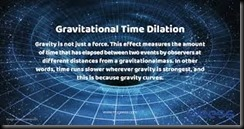However, we also know the gravitational density of the universe has a nonlinear slowing effect on its expansion because its attractive properties decrease as the volume of the universes increase due to its expansion.   In other words, the gravitational density has an opposite effect on its expansion. Therefore, to determine the actual the rate of expansion of the universe at each point in its history one must not only take into account the time dilation due to its gravitational density but also the slowing effect that density has on its expansion at each of those point.  In other words, one makes it APPEAR to move slower while the other faster.

Yet, because of the non-linear effects between the time dilation created by its gravitational density and the effects that density has on the universe rate of expansion there will be a point in its history were one will APPEAR to overtake the other.

The fact that it has been observed that about 4 billion years ago the universe’s expansion change from decelerating to an accelerated phase give us a way to verify the above conclusion

In other words if one calculates the expansion rate of the universe by taking into account the APPARENT speeding up of its evolution resulting from the increase rate at time passes due to the decreasing gravitational density of an expanding universe and finds that it counteracts slowing effect cause by that density about 4 billion years ago it would go a long way to verify the above mechanism.

Some may say that gravitational time dilation would not effect the timing of the expansion because it is  also expanding.   However, Einstein define the time dilation only in terms of the effects a differential gravitational potential has on it therefore an expanding universe will have not effect it.  Some may also say that because the universe is expanding the gravitational density is expanding and weakening at the same rate therefore when we look back the effects it will have on the timing of its expansion will cancel.   However, Einstein tells us the timing of events that cause the universe to expand in the past is locked along with the gravitation density of the universe at the time the expansion took place  Therefore, we must measure the the timing of the events that define the them from the perspective of the gravitational density when those events took place.

Other may say the time dilation effect as discussed in this post is introduced in this calculation, the age of the universe might come down heavily.  This would be true if time was the only factor that must be considered when defining the rate of the universe expansion. For example the gravitational density of the universe would not only effect the passage of time but also it would cause a slowing of expansion due to its attractive forces  Therefore, to define its actual expansion rate one must take into account both slowing effect of gravities attractive forces and the effects it has on time.  The apparent change over to an accelerating universe occurs because of the non-linear relationship that gravity has on time and the expansion rate.  It is possible if one takes both the magnitude of gravity attractive force has on the universe expansion rate and time dilation on its expansion we may discover that it is older than the currently accepted value  of 13 billion years.

<font face="Arial">New Page 1</font>Please visit our Facebook group The Road to unification of Quantum and Relativistic theories if you would like to comment or contribute to our project e Road to unification part  2007 thru 2010  Ebook

 The Road to Unifying QM with Relativity part 1 2007 thru 2010Ebook \$8.00 Paper Back \$15.00 The Road to Unifying QM with Relativity part 2 2011 thru 2014Ebook \$8.00 Paper Back \$16.00 The Road to Unifying QM with Relativity part 3 2015 thru 2020Ebook \$8.00 Paper Back \$18.00

The post Gravity time dilation and the accelerating universe appeared first on Unifying Quantum and Relativistic Theories.

Understanding the dynamics of the uncertainty principle in terms of space time

Quantum mechanics states what the universe is made of while not giving an explanation of why it is that way while Relativity gives us an explanation of why it is what it is but does not tell us what is it made of.  For example, a quantum world is defined in terms of the wave-particle […] The post Understanding the dynamics of the uncertainty principle in terms of space time appeared first on Unifying Quantum and Relativistic...

Quantum mechanics states what the universe is made of while not giving an explanation of why it is that way while Relativity gives us an explanation of why it is what it is but does not tell us what is it made of.  For example, a quantum world is defined in terms of the wave-particle duality of existence while its interactions with that world are defined in terms probabilities and the uncertainty principal which states one cannot precisely measure the properties of Conjugate pairs such as the momentum or position of a particle with complete accuracy.  However, it does not give an explanation of what existence is or how it interacts with its environment to create the universe we live in.  On the other hand, Relativity explains the existence of the universe in terms of an interaction between space and time without telling us what wave-particle duality of existence is or how it interacts with it to create the uncertainty principal.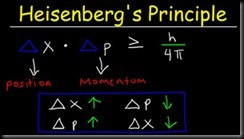However, to understand the dynamics of the uncertainty principle in terms of space-time we must first establish a physical connection between the wave function and the properties of the space-time. This can be accomplished because in Relativity the evolution of space-time is defined in terms of an electromagnetic wave while, as was mentioned earlier the wave function represents how the quantum world evolves to the point where it is observed.

This commonality suggests the wave function could be represented by an electromagnetic wave in space-time.  This means to derive the wave particle duality of existence defined by the wave function in terms of space-time one must physically connect the evolution of an electromagnetic wave to its existence.

This is possible because the science of wave mechanics and Relatively tells us an electromagnetic wave moves continuously through space-time unless it is prevented from moving through time by someone or something interacting with it. This would result in it being confined to three-dimensional space. The science of wave mechanics also tells us the three-dimensional "walls" of this confinement will result in its energy being reflected back on itself thereby creating a resonant or standing wave in three-dimensional space. This would cause the energy of an electromagnetic wave to be concentrated at the point in space were a particle would be found.  Additionally, wave mechanics also tells us the energy of a resonant system such as a standing wave can only take on the discrete or quantized values associated with its fundamental or a harmonic of its fundamental frequency.

For example, it explains why if the wave function is prevented from evolving through the probability field that defines a quantum existence by an observation it presents itself as a particle in terms of the properties of an electromagnetic wave in a space-time environment.

Yet, it also tells us, similar to the evolution of an electromagnetic wave in space-time if unobserved the it will continue evolve through the probabilistic universe defined by quantum mechanics.

In other words, it shows how one can understand the evolution of wave-particle duality of a quantum existence by comparing it to the evolution of an electromagnetic wave in space-time

Next, we must explain how quantum mechanics definition of a particle in terms of a one-dimensional point is responsible for the validity of the uncertainty principal.

Relativity and the science of wave mechanics tell us the energy of the standing wave which earlier defined a particle would be distributed over a volume of space-time that corresponds to is wavelength. However, to accurately determine its momentum or position one must be able to determine where those measurement are taken with respect to energy volume those system occupy.

Yet, to measure momentum of a particle in the quantum world one must determine time it takes to move between two points in the probability field.  Therefore, they will be an inherent uncertainty if one cannot determine where with respect to volume of the system those points are.

But because in Relativity the measurement of position is taken with respect to its spatial not its time properties one should define it with respect to space not time

Einstein gave us the ability to do this when he defined the mathematical relationship between space and time in terms of the constant velocity of light because in doing so, he provided a method of converting a unit of time in a space-time environment to its equivalent unit of space in four *spatial* dimensions.  Additionally, because the velocity of light is constant, he also defined a one to one quantitative and qualitative correspondence between his space-time universe and one made up of only four *spatial* dimensions.

In other words, one can use a displacement in the "surface" of three-dimensional space with respect to a fourth *spatial* dimension to define a particles position while using a displacement with respect to a time dimension to define its momentum.

As was mentioned earlier Einstein defined the energy volume of a system in terms of a displacement in space-time and because energy can neither be created or destroyed it will remain constant therefore the volume of the displacement in space time will also remain constant.

HOWEVER, BECAUSE QUANTUM MECHANICS IS INFORMATION BASED ONE CAN SAY THE INFORMATION VOLUME OF A SYSTEM ALSO REMAINS CONSTANT.

Therefore, because the measurement of monument or position does not change the energy of a system the combined information volume associated with momentum and the displacement associated with position remains constant.

As was also mentioned earlier Quantum mechanics defines both moment and position with respect to a one-dimensional point in a probability field.  However, the accuracy of the information as to where that point is in relation to its information volume is directly related to how much information is taken from the system.  In other words, the more accurate the measurement the more information regarding it must be removed from the system.

However, because, as was mentioned earlier the information volume of a system remains constant the more information taken out of it regarding its momentum will result in there being more space for the point defining its position to occupy. This makes the determination of its position more uncertain because it could be found in anywhere in that larger volume.

This shows how one can define a dynamic relationship between the uncertainty of determining the momentum or position of a particle in terms of interaction between space and time and why it is responsible for the uncertainty principal.  This is because it defines why in terms of the properties of space-time the more information you have about the momentum of a particle the less you can know about it position and the more information you have about its position the less you can know about its momentum.

In other words, it shows how a dynamic interaction between the spatial and time properties of the universe can not only explain the particle wave duality of existence but also the uncertainty principal of quantum mechanics

Copyright Jeffery B O’Callaghan Nov. 2020

<font face="Arial">New Page 1</font>Please visit our Facebook group The Road to unification of Quantum and Relativistic theories if you would like to comment or contribute to our project e Road to unification part  2007 thru 2010  Ebook

 The Road to Unifying QM with Relativity part 1 2007 thru 2010Ebook \$8.00 Paper Back \$15.00 The Road to Unifying QM with Relativity part 2 2011 thru 2014Ebook \$8.00 Paper Back \$16.00 The Road to Unifying QM with Relativity part 3 2015 thru 2020Ebook \$8.00 Paper Back \$18.00

Why the future is what it is

Classical physics is causal; complete knowledge of the past allows for the computation of the future. Likewise, complete knowledge of the future allows precise computation of the past. Not so in Quantum Physics. Objects are neither particles nor waves; they are a strange combination of both. Given complete knowledge of the past, we can make […] The post Why the future is what it is appeared first on Unifying Quantum and Relativistic...

Classical physics is causal; complete knowledge of the past allows for the computation of the future. Likewise, complete knowledge of the future allows precise computation of the past.

Not so in Quantum Physics. Objects are neither particles nor waves; they are a strange combination of both. Given complete knowledge of the past, we can make only probabilistic predictions of the future.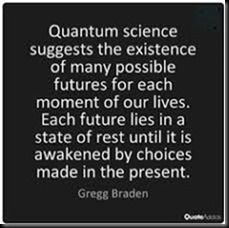In other words, classical mechanics tells us that only one future exists while quantum mechanics tells us that due to its probabilistic interpretation of wave-particle duality of existence, many different ones simultaneously exist and which one become a reality is determined by observation.  Additionally, it states that they are randomly disturbed throughout existence.

On the surface these probabilistic and causal definitions of the future appear to incompatible.

However, that may not be the case.

As mentioned earlier, one of the things that separate the future associated with classical physics from probabilistic one of quantum mechanical is one tell us all of the probable future outcomes of an observation exist while the other which based on causality tells us there in only one.

However, when we role dice in a casino most do not think there are six of them out there waiting for the dice to tell us which one we will occupy after the roll.  This is because the probability of getting a six is related to its physical interaction with properties of the table in the casino where it is rolled. This means the probability of getting a six is determined by the physical properties of the dice and the casino it occupies.  In other words, the probabilities associated with a roll of the dice does not define the future of the casino the casino defines the future of the dice.

Similarly, just because Quantum mechanics defines outcome of an observation in terms of probabilities would not mean all the of the predicted futures exist if the probability of a specific outcome is caused by a physical interaction of the wave-particle duality of existence with the universe it occupies.  In other words, like the dice, it is possible the wave-particle duality of existence does not define the future of the universe the universe defines the future of its wave-particle component.

However, to understand how this is possible one would have to show the probability of a specific outcome of an observation is related to the interaction of the wave-particle duality of existence and the space it occupies.

For example, in the article “Why is energy/mass quantized?” Oct. 4, 2007 it was shown the wave-particle duality of existence defined by quantum mechanics can be derived by extrapolating the laws of classical resonance in a three-dimensional environment to an energy wave on a "surface" of a three-dimensional space manifold with respect to a time dimension.

Briefly it showed the four conditions required for resonance to occur in a classical environment, an object, or substance with a natural frequency, a forcing function at the same frequency as its natural frequency, the lack of a damping frequency and the ability for the substance to oscillate spatial would occur in an environment consisting of four-dimensional space-time.

The existence of four-dimensional space-time would give an energy wave the ability to oscillate spatially on a "surface" the third spatial dimension with respect to the time dimension thereby fulfilling one of the requirements for classical resonance to occur.

These oscillations would be caused by an event such as the decay of a subatomic particle or the shifting of an electron in an atomic orbital. This would force the "surface" of a three-dimensional space manifold to oscillate with the frequency associated with the energy of that event.

The oscillations caused by such an event would serve as forcing function allowing a resonant system or "structure" to be established space.

Therefore, these oscillations in a "surface" of a three-dimensional space manifold would meet the requirements mentioned above for the formation of a resonant system or "structure" in four-dimensional space-time if one extrapolated them to that environment.

Classical mechanics tells us the energy of a resonant system can only take on the discrete or quantized values associated with its fundamental or a harmonic of its fundamental frequency.

Hence, these resonant systems in four-dimensional space-time would be responsible for particle property of existence in the space-time environment of Einstein.

Yet one can also define the boundary conditions responsible for a creating the resonant system or "structure" that earlier defined a particle.

For example, in our three-dimensional world, a point on the two-dimensional surface of paper is confined to that surface. However, that surface can oscillate up or down with respect to three-dimensional space.

However, the edge of the paper provides a boundary that reflects those oscillation back on itself, thereby creating a resonant wave on the surface of the paper.

Similarly, an energy wave of three-dimensional space would be confined to it however, it could, similar to the surface of the paper oscillate “up” or “down” while moving through time.

However, when it is prevented from moving thought time either by being observed or encountering an object or particle that wave energy will be reflected back on itself, thereby creating a resonant wave on the "surface" three-dimensional space,

In other words, if the wave component of quantum existence is prevented from moving unhindered through time either by an observation or by an interaction with a particle or object it will create a resonant system or structure that defined the quantum properties of existence in the article "Why is energy/mass quantized?".

This shows how, one can derive the wave-particle duality of quantum existence in terms of an interaction of space with time.

The final step in answering the question of why the future is what it is requires one to show how the quantum mechanical wave-particle duality of existence interacts with space to create the future in terms of probabilities.

One can use the analogy of energy of a vibrating or oscillating ball on a rubber diaphragm and how the magnitude of those vibrations would would be greatest at the focal point of those vibrations and decrease as one move away from them.

Similarly, if the assumption that wave properties of a quantum existence represents vibrations or oscillations in a "surface" of three-dimensional space, is correct the magnitude of those oscillations would be greatest at the focal point of and decreases as one moves away from it.

However, as the article, mentioned earlier “Why is energy/mass quantized?” showed the particle property of existence is a result of a resonant structure formed on the "surface" of a three-dimensional space manifold by its interaction with the time dimension.

Yet the science of Wave Mechanics tells us resonance would most probably occur on the surface of the rubber sheet were the magnitude of the vibrations is greatest and would diminish as one move away from that point.

Similarly, the resonant structure that article associated with a particle property of existence would most probably be found were the magnitude of the vibrations in a "surface" of a three-dimensional space manifold is greatest and would diminish as one move away from that point.

In other words, one can define the future of the quantum mechanical wave-particle duality of existence in terms a causal interaction between it and the universe it occupies.

Additionally, this shows why defining the outcome of an observation of the wave-particle duality of existence as quantum mechanics does in terms of probabilities does not mean all the of those predicted futures exist.  This is because similar to the dice mentioned earlier the probability of a specific future is caused by a physical interaction of it with the universe it occupies.

In other words, the reason why the future is what it is is because the wave-particle duality of existence does not define the future of the universe the universe defines the future of its wave-particle component.

Later Jeff

<font face="Arial">New Page 1</font>Please visit our Facebook group The Road to unification of Quantum and Relativistic theories if you would like to comment or contribute to our project e Road to unification part  2007 thru 2010  Ebook

 The Road to Unifying QM with Relativity part 1 2007 thru 2010Ebook \$8.00 Paper Back \$15.00 The Road to Unifying QM with Relativity part 2 2011 thru 2014Ebook \$8.00 Paper Back \$16.00 The Road to Unifying QM with Relativity part 3 2015 thru 2020Ebook \$8.00 Paper Back \$18.00

The post Why the future is what it is appeared first on Unifying Quantum and Relativistic Theories.

The Physics of Quantum Gravity

There are two ways science attempts to understand or define the behavior of our world. The first is Quantum mechanics or the branch of physics that uses probabilities to define the wave particle duality of existence.  The other is the deterministic universe of Einstein where the interactions of space with time determines the casualty of […] The post The Physics of Quantum Gravity appeared first on Unifying Quantum and Relativistic...

There are two ways science attempts to understand or define the behavior of our world. The first is Quantum mechanics or the branch of physics that uses probabilities to define the wave particle duality of existence.  The other is the deterministic universe of Einstein where the interactions of space with time determines the casualty of events in the macroscopic universe we live in.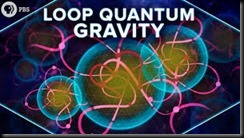Specifically, the General Theory of Relativity tells us that gravity is a result of a curvature in space-time whose magnitude is directly related to the amount of matter or number of particles contained in given volume of space-time.

While quantum mechanics use probabilistic properties of the wave particle duality of existence to define the position of particles such as protons and elections in a given volume of space.

Since we all live in the same world you would expect the probabilistic approach of quantum mechanics to be compatible with the deterministic one of Einstein.  Unfortunately, they define two different worlds that on the surface appear to be incompatible.  One defines existence in terms of the probabilities associated with the wave particle duality of existence mentioned earlier while the other defines it in terms the deterministic of properties the continuous field of space and time.

Therefore, one could argue the Physicist of Quantum Gravity is the science of explaining the how the probabilities associated the wave particle duality of a quantum existence controls the deterministic universe of gravity as defined by Einstein or show how that universe is responsible for those probabilities in terms of the interaction of space and time.

For example, one can derive the probability of getting a six on a role of dice based on the fact that dice physically has six sides with only one having a six on it.  This is true even though one cannot predict when a six will occur.  In other words. one can show that probability getting a six on the role of a dice is determined by the physical properties of the dice and not that the probably of six occurring is the reason one rolls a six.

Similarly, if one can show the position of a particle is not caused by the probability of finding it there but is caused by a property of space-time one could understand how to connect gravity with the probabilistic world of quantum mechanics.  This is because, as mentioned earlier Einstein defined gravity in terms of the number of particles in given volume of space-time.

For example, in the article “Why is energy/mass quantized?” Oct. 4, 2007 it was shown the wave particle duality of existence define by quantum mechanics can be understood by extrapolating the laws of classical resonance in a three-dimensional environment to an energy wave on a "surface" of a three-dimensional space manifold with respect to a time dimension.

Briefly it showed the four conditions required for resonance to occur in a classical environment, an object, or substance with a natural frequency, a forcing function at the same frequency as its natural frequency, the lack of a damping frequency and the ability for the substance to oscillate spatial would occur in an environment consisting of four-dimensional space-time.

The existence of four-dimensional space-time would give an energy wave the ability to oscillate spatially on a "surface" the third spatial dimension with respect to the time dimension thereby fulfilling one of the requirements for classical resonance to occur.

These oscillations would be caused by an event such as the decay of a subatomic particle or the shifting of an electron in an atomic orbital.  This would force the "surface" of a three-dimensional space manifold to oscillate with the frequency associated with the energy of that event.

The oscillations caused by such an event would serve as forcing function allowing a resonant system or "structure" to be established space.

Therefore, these oscillations in a "surface" of a three-dimensional space manifold would meet the requirements mentioned above for the formation of a resonant system or "structure" in four-dimensional space-time if one extrapolated them to that environment.

Classical mechanics tells us the energy of a resonant system can only take on the discrete or quantized values associated with its fundamental or a harmonic of its fundamental frequency.

Hence, these resonant systems in four-dimensional space-time would be responsible for particle property of existence in the space-time environment of Einstein.

Yet one can also define the boundary conditions responsible for a creating the resonant system or "structure" that earlier defined a particle.

For example, in our three-dimensional world, a point on the two-dimensional surface of paper is confined to that surface.  However, that surface can oscillate up or down with respect to three-dimensional space.

However, the edge of the paper provides a boundary that reflects those oscillation back on itself, thereby creating a resonant wave on the surface of the paper.

Similarly, an energy wave of three-dimensional space would be confined to it however, it could, similar to the surface of the paper oscillate “up” or “down” while moving through time.

However, when it is prevented from moving thought time either by being observed or encountering an object or particle that wave energy will be reflected back on itself, thereby creating a resonant wave on the "surface" three-dimensional space,

In other words, if the wave component of quantum existence is prevented from moving unhindered through time either by an observation or by an interaction with a particle or object it will create a resonant system or structure that defined the quantum properties of existence in the article "Why is energy/mass quantized?".

This shows how, one can explain the wave particle duality quantum existence in terms of an interaction of space with time.

The final step in integrating quantum mechanics with Einstein gravitational theories is to physical connect its probabilistic interoperation of a particles position with the physical properties of space-time as defined by him.

The physics of wave mechanics tells us, due to the continuous properties the energy wave of a quantum system  means it would be distributed throughout "surface" a three-dimensional space manifold with respect to time.

For example, the energy of a vibrating or oscillating ball on a rubber diaphragm would be disturbed over its surface while the magnitude of those vibrations would decrease as one move away from the focal point of the oscillations.

Similarly, if the assumption that wave properties of a quantum existence represents vibrations or oscillations in a "surface" of three-dimensional space, is correct these oscillations would be distributed over the "surface" three-dimensional space while the magnitude of those vibrations would be greatest at the focal point of the oscillations and decreases as one moves away from it.

However as the article, mentioned earlier  “Why is energy/mass quantized?” showed the particle property of existence is a result of a resonant structure formed on the "surface" of a three-dimensional space manifold by its interaction with the time dimension.

Yet the science of Wave Mechanics tells us resonance would most probably occur on the surface of the rubber sheet were the magnitude of the vibrations is greatest and would diminish as one move away from that point.

Similarly, the resonant structure that article associated with a particle properties of existence would most probably be found were the magnitude of the vibrations in a "surface" of a three-dimensional space manifold is greatest and would diminish as one move away from that point.

This explains why in terms of the physical properties of four-dimensional space-time why one must use the probabilities associate with quantum mechanics to determine the exact the position a single particle in space.  However, it allows one integrate the probabilities associated with the quantum mechanical definition of existence with the physical properties gravity because as was mentioned earlier gravity can be defined in terms of the quantity of particles in a given volume of space-time.

In other words, the gravitational force in a given region of space-time will be greater where the probability density of particles as define by quantum mechanics is the greatest.

As was mentioned earlier one can show that probability getting a six on the role of a dice is determined by the physical properties of the dice and not that the probably of six occurring is the reason one rolls a six.

In other word, the Physicists of Quantum Gravity may not be related to the quantum probability of finding a particle in a given region of space-time but to determining reasons why those probabilities are what they are.

Later Jeff

<font face="Arial">New Page 1</font>Please visit our Facebook group The Road to unification of Quantum and Relativistic theories if you would like to comment or contribute to our project e Road to unification part  2007 thru 2010  Ebook

 The Road to Unifying QM with Relativity part 1 2007 thru 2010Ebook \$8.00 Paper Back \$15.00 The Road to Unifying QM with Relativity part 2 2011 thru 2014Ebook \$8.00 Paper Back \$16.00 The Road to Unifying QM with Relativity part 3 2015 thru 2020Ebook \$8.00 Paper Back \$18.00

The post The Physics of Quantum Gravity appeared first on Unifying Quantum and Relativistic Theories.